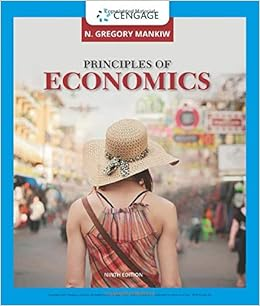# Exhibit policy interaction based on the graph

• Test Prep
• 38
• 86% (7) 6 out of 7 people found this document helpful

This preview shows page 10 - 12 out of 38 pages.

##### We have textbook solutions for you!
The document you are viewing contains questions related to this textbook.The document you are viewing contains questions related to this textbook.
Chapter 26 / Exercise 5
Principles of Economics
MankiwExpert Verified
38. (Exhibit: Policy Interaction) Based on the graph, starting from equilibrium at interest rate r3, income Y2, IS1, and LM1, if there is an increase in government spending that shifts the IScurve to IS2and the central bank does not change the money supply, the new equilibrium combination of interest and income will be: A) r1, YB) r2, YC) r3, YD) r3, Y
2.
3.
3.
4.39. Over the last 25 years, the estimates of the one-year expenditure multiplier that have been calculated from macroeconometric models of Canada have fallen from about ______ to about _______.
40. Over the last 25 years, the estimates of the one-year expenditure multiplier that have been calculated from macroeconometric models of Canada have fallen because I: economists have made major progress in their ability to model and simulate how individuals form expectations; II: exchange-rate changes have become increasingly important.
41. An increase in investment demand for any given level of income and interest ratesdue, for example, to more optimistic animal spirits”—will, within the ISframework, ______ output and ______ interest rates. LM
42. An increase in household saving for any given level of income will shift the: A) LMcurve upward and to the left. B) LMcurve downward and to the right. C) IScurve downward and to the left. D) IScurve upward and to the right.
##### We have textbook solutions for you!
The document you are viewing contains questions related to this textbook.The document you are viewing contains questions related to this textbook.
Chapter 26 / Exercise 5
Principles of Economics
MankiwExpert Verified
Page 11 43. An increase in the demand for money, at any given income level and level of interest rates, will, within the ISLMframework, ______ output and ______ interest rates.
44. In the ISLMmodel, a decrease in the interest rate would be the result of a(n):
45. In the ISLMmodel, a decrease in output would be the result of a(n):
46. When bond traders for the Bank of Canada seek to increase interest rates, they ______ bonds, which shifts the ______ curve to the left. A) buy; B) buy; C) sell; D) sell;
IS
LM
IS
LM47. When bond traders for the Bank of Canada seek to decrease interest rates, they ______ bonds, which shifts the ______ curve to the right.
IS
LM
IS
LM
•••